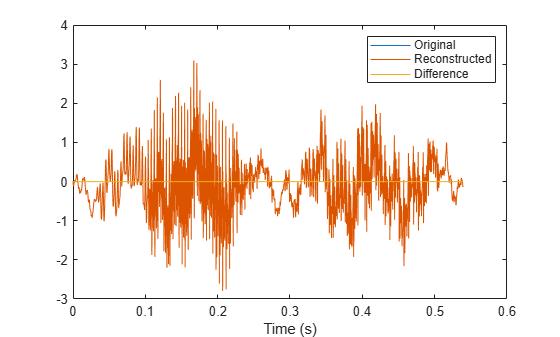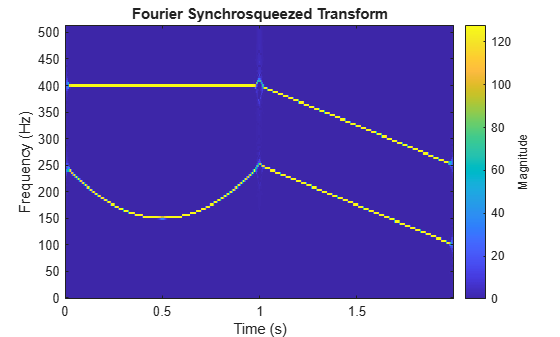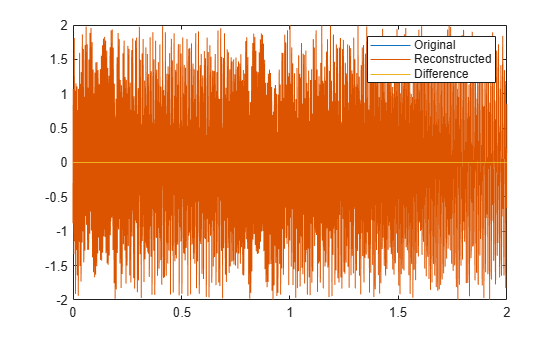# ifsst

Inverse Fourier synchrosqueezed transform

## Syntax

``x = ifsst(s)``
``x = ifsst(s,window)``
``x = ifsst(s,window,f,freqrange)``
``x = ifsst(s,window,iridge)``
``x = ifsst(s,window,iridge,'NumFrequencyBins',nbins)``

## Description

example

````x = ifsst(s)` returns the inverse Fourier synchrosqueezed transform of `s`. `x` is reconstructed using the entire time-frequency plane in `s`.```

example

````x = ifsst(s,window)` reconstructs the signal whose Fourier synchrosqueezed transform was computed using `window`.```
````x = ifsst(s,window,f,freqrange)` inverts the synchrosqueezed transform assuming it was sampled at the frequencies `f`, which lie within `freqrange`. The synchrosqueezed transform is inverted for the bins in `s` whose frequencies are within `freqrange`.```

example

````x = ifsst(s,window,iridge)` inverts the synchrosqueezed transform along the time-frequency ridges specified by the index vector or matrix `iridge`. If `iridge` is a matrix, then `ifsst` initially performs the inversion along the first column of `iridge` and then proceeds iteratively along the subsequent columns. The output is a vector or matrix with the same size as `iridge`.```
````x = ifsst(s,window,iridge,'NumFrequencyBins',nbins)` specifies the number of frequency bins around the indices in `iridge` to use in the reconstruction.```

## Examples

collapse all

Load a speech signal sampled at ${F}_{s}=7418\phantom{\rule{0.2777777777777778em}{0ex}}Hz$. The file contains a recording of a female voice saying the word "MATLAB®." Compute the Fourier synchrosqueezed transform of the signal.

```load mtlb % To hear, type sound(mtlb,Fs) [sst,f] = fsst(mtlb,Fs);```

Invert the transform to reconstruct the signal. Plot the original and reconstructed signals, as well as the difference between them.

```xrec = ifsst(sst); t = (0:length(mtlb)-1)/Fs; plot(t,mtlb,t,xrec,t,mtlb-xrec) xlabel('Time (s)') legend('Original','Reconstructed','Difference')```Check the accuracy of the reconstruction by computing the ${\ell }_{\infty }$ norm of the difference between the original signal and the inverse transform.

`Linf = norm(abs(mtlb-xrec),Inf)`
```Linf = 1.9762e-14 ```
`% To hear, type sound(mtlb-xrec,Fs)`

Generate a signal sampled at 1024 Hz for 2 seconds.

```nSamp = 2048; Fs = 1024; t = (0:nSamp-1)'/Fs;```

During the first second, the signal consists of a 400 Hz sinusoid and a concave quadratic chirp. Specify a chirp that is symmetric about the interval midpoint, starts and ends at a frequency of 250 Hz, and attains a minimum of 150 Hz.

```t1 = t(1:nSamp/2); x11 = sin(2*pi*400*t1); x12 = chirp(t1-t1(nSamp/4),150,nSamp/Fs,1750,'quadratic'); x1 = x11+x12;```

The rest of the signal consists of two linear chirps of decreasing frequency. One chirp has an initial frequency of 250 Hz that decreases to 100 Hz. The other chirp has an initial frequency of 400 Hz that decreases to 250 Hz.

```t2 = t(nSamp/2+1:nSamp); x21 = chirp(t2,400,nSamp/Fs,100); x22 = chirp(t2,550,nSamp/Fs,250); x2 = x21+x22;```

Compute the Fourier synchrosqueezed transform of the signal. Specify a 256-sample Kaiser window with a shape parameter β = 100. Use the plotting functionality of `fsst` to display the result.

```sig = [x1;x2]; wind = kaiser(256,120); [sigtr,ftr,ttr] = fsst(sig,Fs,wind); fsst(sig,Fs,wind,'yaxis')```Invert the transform to reconstruct the function. Plot the original and inverted signals and the difference between them.

```x = ifsst(sigtr,wind); plot(t,sig,t,x,t,x-sig) legend('Original','Reconstructed','Difference')````diffnorm = norm(x-sig)`
```diffnorm = 3.9031e-13 ```

Generate a signal that consists of two chirps. The signal is sampled at 3 kHz for one second. The first chirp has an initial frequency of 400 Hz and reaches 800 Hz at the end of the sampling. The second chirp starts at 500 Hz and reaches 1000 Hz at the end. The second chirp has twice the amplitude of the first chirp.

```fs = 3000; t = 0:1/fs:1-1/fs; x1 = chirp(t,400,t(end),800); x2 = 2*chirp(t,500,t(end),1000);```

Compute and plot the Fourier synchrosqueezed transform of the signal. Display the time on the x-axis and the frequency on the y-axis.

```[sst,f] = fsst(x1+x2,fs); fsst(x1+x2,fs,'yaxis')```Extract the ridge corresponding to the higher-energy component of the signal, which is the chirp with the larger amplitude. Use the ridge to reconstruct the signal.

```[~,iridge] = tfridge(sst,f); xrec = ifsst(sst,[],iridge);```

Plot the spectrogram for the higher-energy component. Divide the component into 256-sample sections and specify an overlap of 255 samples. Use 512 DFT points and a rectangular window.

`spectrogram(xrec,rectwin(256),255,512,fs,'yaxis')`To extract the second chirp, specify that `tfridge` search for two ridges. The second column of the output is the lower-energy component of the signal.

```[~,iridge] = tfridge(sst,f,'NumRidges',2); xrec = ifsst(sst,[],iridge(:,2)); spectrogram(xrec,rectwin(256),255,512,fs,'yaxis')```## Input Arguments

collapse all

Input synchrosqueezed transform, specified as a matrix.

Example: `fsst(cos(pi/4*(0:159)))` specifies the synchrosqueezed transform of a sinusoid.

Data Types: `single` | `double`
Complex Number Support: Yes

Spectral window, specified as an integer or as a row or column vector.

• If `window` is an integer, then `ifsst` assumes that the synchrosqueezed transform, `s`, was computed using a Kaiser window of length `window` and β = 10.

• If `window` is a vector, then `ifsst` assumes that `s` was computed by windowing each segment of the original signal using `window`.

• If `window` is not specified, then `ifsst` assumes that `s` was computed using a Kaiser window of length 256 and β = 10. If the signal to be reconstructed, `x`, has fewer than 256 samples, then you must provide a window length or window vector consistent with the length of `x`.

For a list of available windows, see Windows.

Example: `hann(N+1)` and `(1-cos(2*pi*(0:N)'/N))/2` both specify a Hann window of length `N` + 1.

Data Types: `double` | `single`

Sampling frequencies, specified as a vector. The length of `f` must equal the number of elements in `s`.

Data Types: `single` | `double`

Frequency range, specified as a two-element vector. The values of `freqrange` must be strictly increasing and must lie in the range comprised by `f`.

Data Types: `single` | `double`

Time-frequency ridge indices, specified as a vector or matrix. `iridge` is an output of `tfridge`.

Data Types: `single` | `double`

Number of neighboring bins on either side of the time-frequency ridges of interest, specified as the comma-separated pair consisting of `'NumFrequencyBins'` and a positive integer scalar. Indices close to the frequency edges that have fewer than `nbins` bins on one side are reconstructed using a smaller number of bins.

Data Types: `single` | `double`

## Output Arguments

collapse all

Inverse synchrosqueezed transform, returned as a vector. The length of `x` equals the number of columns in `s`.